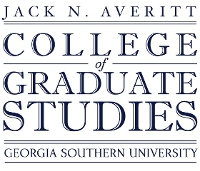## Electronic Theses and Dissertations

#### Title

Gallai-Ramsey Number for Classes of Brooms

Spring 2019

#### Degree Name

Master of Science in Mathematics (M.S.)

#### Document Type and Release Option

Thesis (open access)

#### Department

Department of Mathematical Sciences

Hua Wang

Colton Magnant

Goran Lesaja

Daniel Gray

#### Committee Member 3 Email

dagray@georgiasouthern.edu

#### Abstract

Given a graph $G$, we consider the problem of finding the minimum number $n$ such that any $k$ edge colored complete graph on $n$ vertices contains either a rainbow colored triangle or a monochromatic copy of the graph $G$, denoted $gr_k(K_{3}:G)$. More precisely we consider $G=B_{m,\ell}$ where $B_{m,\ell}$ is a broom graph with $m$ representing the number of vertices on the handle and $\ell$ representing the number of bristle vertices. We develop a technique to reduce the difficulty of finding $gr_{k}(K_{3}:B_{m,\ell})$, and use the technique to prove a few cases with a fixed handle length, but arbitrarily many bristles. Further, we find upper and lower bounds for any broom.

1102321994

No

COinS